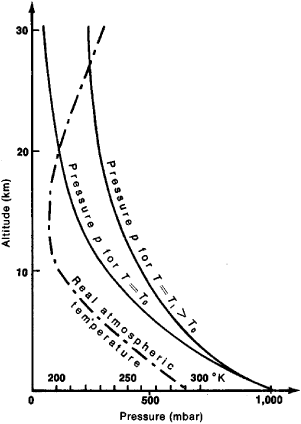# Barometric Formula

Also found in: Wikipedia.

## Barometric Formula

a formula that determines the dependence of the pressure or density of a gas on the altitude in a gravitational field.

For an ideal gas that has a constant temperature T and is located in a uniform gravitational field (the free-fall acceleration g is the same at all points), the barometric formula has the form

p = p0 exp[– gμ (hh0)/RT]

where p is the pressure of the gas in a layer located at the altitude h, p0 is the pressure at the zero level (h = h0), μ is the molecular mass of the gas, R is the gas constant, and T is the absolute temperature. The above dependence is represented graphically in Figure 1. It follows from the above barometric formula that the concentration of molecules n (or the gas density) decreases with altitude according to the same law:

n = n0 exp[–mg (hh0)/kT]

where m is the mass of the molecule and k is Boltzmann’s constant.T)."" height="424" width="301"/>
Figure 1. Decrease in pressure of a gas in relation to altitude in a uniform gravitational field at a constant gas temperature (Tˌ > T). The dotted curve indicates the real variation of atmospheric temperature with altitude.

The barometric formula can be obtained from the law of velocity and coordinate distribution of the molecules of an ideal gas in a potential force field. Two conditions—constancy of gas temperature and uniformity of force field—must be satisfied in this situation. Analogous conditions can be satisfied for minute solid particles that are suspended in a liquid or gas. Based on this, the French physicist J. Perrin in 1908 applied the barometric formula to the height distribution of the particles of an emulsion, allowing him to determine directly the Boltzmann constant.

The barometric formula shows that the density of a gas decreases exponentially with altitude. The quantity –mg (hh0)/kT, which determines the rate of decrease of the density, is the ratio of the potential energy of the particles to their average kinetic energy, which is proportional to kT. The higher the temperature T, the slower the decrease of density with altitude. On the other hand, the increase of the force of gravity mg (at a constant temperature) leads to a considerably larger compression of the lower layers and to an increase of the pressure drop (gradient). The gravitational force mg that acts on the particles can change on account of two quantities—the acceleration g and the mass m. Consequently, in a mixture of gases that is located in a gravitational field, molecules of different mass are distributed at different altitudes.

The real distribution of air pressure and density in the earth’s atmosphere does not obey the barometric formula, since within the boundaries of the atmosphere the temperature and acceleration of free fall vary with altitude and geographic latitude. In addition, the atmospheric pressure increases with the concentration of water vapor in the atmosphere.

The barometric formula is the basis for barometric altimetry—a method of determining the difference in altitude Δh between two points from the pressures (pi and p2) measured at these points. Inasmuch as the atmospheric pressure depends on the weather, the time interval between measurements must be as small as possible, and the measurement points must not be too far apart. In this case the barometric formula is written in the form Δh = 18,400(1 + ɑt)log(p1/p2) (in meters), where t is the average temperature of the layer of air between the measuring points and α is the temperature coefficient of the volumetric expansion of air. The error for calculations with this formula does not exceed 0.1–0.5 percent of the altitude being measured. The Laplace formula, which takes into account the effect of air humidity and the variation of the acceleration of free fall, is more accurate.

### REFERENCE

Khrgian, A. Kh. Fizika atmosfery. Moscow, 1958.

IU. N. DROZHZHIN

Site: Follow: Share:
Open / Close